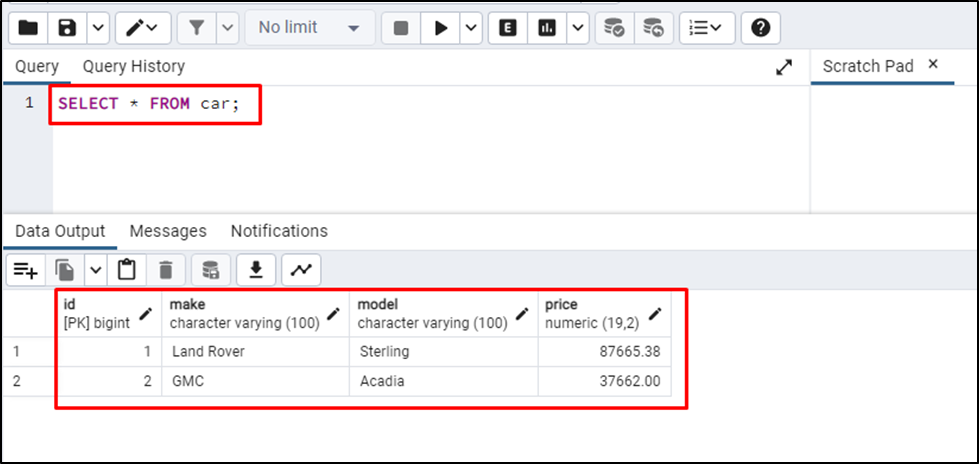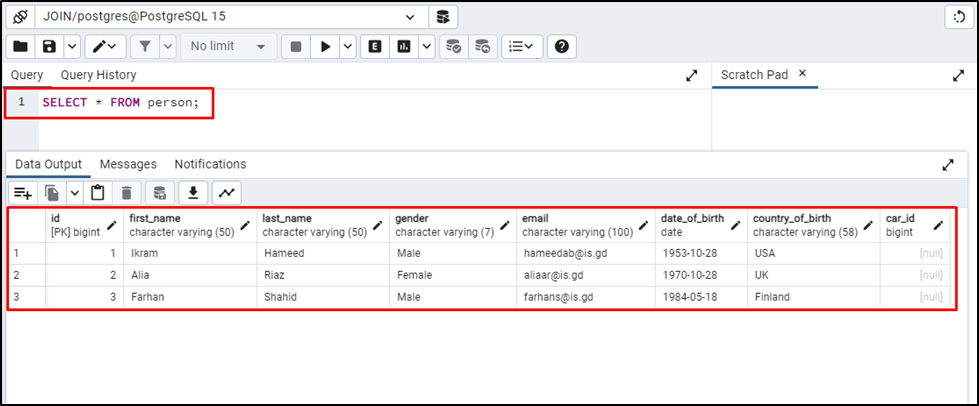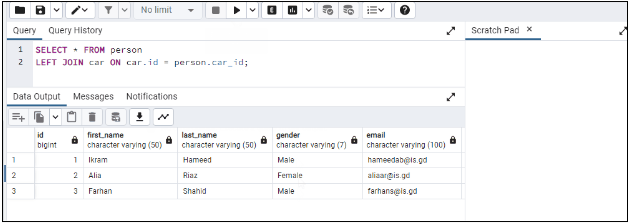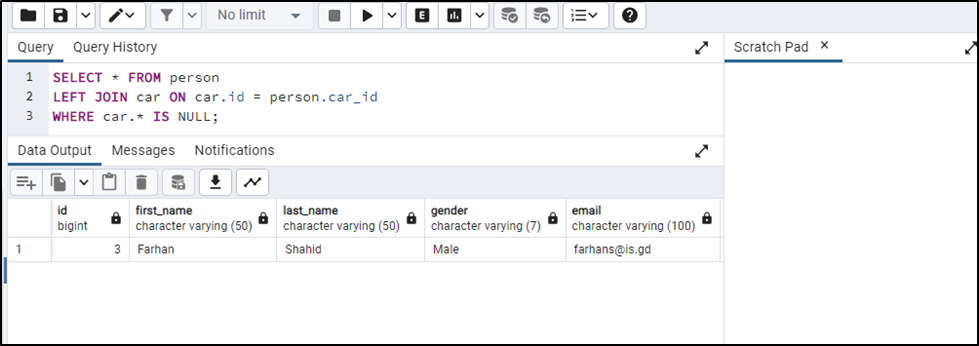##### How Does LEFT JOIN Work With PostgreSQL

JOINs hold a very important place in the field of the database as they can be used to combine multiple tables on the DB. They are used in both relational and sequel databases as they carry the same importance in both types of databases. LEFT JOIN is the type of JOINs that combines tables and returns all data from the left table and gets only the corresponding records of the right table.

This guide will explain the working of LEFT JOIN with PostgreSQL.

How Does LEFT JOIN Work With PostgreSQL?

LEFT JOIN is used in the PostgreSQL database to return data from the left table and corresponding records from the right table. For instance, “A” is the left table and “B” is the right table, applying LEFT JOIN will return all the records from table A and only matched ones from table B.

Syntax

The following code block contains the syntax to apply the LEFT JOIN in the PostgreSQL database:

```SELECT <Columns>
FROM <TableA>
LEFT JOIN <TableB>
ON TableA.Column_x = TableB.Column_y;```

The above code suggests:

- SELECT is a statement used to choose one or more Columns of the given table and the “*” is used to select all columns from the table which in this case is the left table or table A.
- The LEFT JOIN keeps the name of the table to be joined with the “TableA”.
- The ON keyword is used to define the joining condition.

Example 1: Combine Tables Using LEFT JOIN

The following example takes two tables from the PostgreSQL database and applies LEFT JOIN to them. This query will be used to get all the data from the “car” table as it uses “*” for columns to get the complete table:

`SELECT * FROM car;`

The above query returns all the data and columns available on the car table:Another sample table named “person” is created with different records. Execute the given query to get all its records:

`SELECT * FROM person;`

Running the above query return the data from the person table:SideNote: The last column which is the “car_id” in the above snippet acts as the foreign key of the car table.

The following query is used to apply LEFT JOIN:

```SELECT * FROM person
LEFT JOIN car ON car.id = person.car_id;```

Here in the above code snippet:

- The SELECT query is used to fetch all the data from the person table based on the PK and FK which is the car_id column.
- The condition for the LEFT JOIN is defined using the ON keyword which takes the id column from the car table:The output shows that the result set contains all the records of the person table and matching records of the car table.

Example 2: Using WHERE Clause With LEFT JOIN

Use the following query to return only null values in the “car” table indicating that they don't have a car:

```SELECT * FROM person
LEFT JOIN car ON car.id =   person.car_id
WHERE car.* IS NULL;```

The above code contains one additional condition to the previous query which goes like this:

- Select all records from the person table and apply LEFT JOIN on the car table.
- The WHERE condition limits the returning record as it only asks for the record that doesn’t have a value in the car table:That’s all about the working of the LEFT JOIN with the PostgreSQL database.

Conclusion

In PostgreSQL, LEFT JOIN is used to return all data from Table A or the Left only the matched values from the right table or Table B. The ON keyword is used with the LEFT JOIN to define the joining condition. Moreover, the WHERE clause can be used with the LEFT JOIN to combine the filtered data. This guide has explained the working of the LEFT JOIN in PostgreSQL using suitable examples.